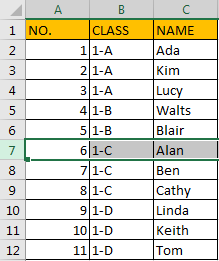# How to Update Sequence Number for Rows Automatically After Inserting or Deleting Rows in Excel

Sometimes we add sequence number in the first column for each row in a table, for example create a table for saving student info, and one student one row. If I insert a new student info or delete an existing student info, the sequence numbers for rows are cut off and not continuous anymore. If we want to make these numbers are still continuous automatically after inserting or deleting rows, do you have any tricks? Actually, there are some methods you can follow to update sequence number for rows automatically, this article will introduce you two methods to implement this.

Precondition:

See table below. In first column, there are sequence numbers for rows.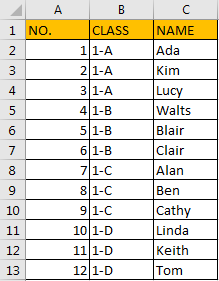If we insert a new row or delete an existing row from the table, obviously numbers are not continuous. So, we need to know the method to update sequence number automatically.

## Method 1: Update Sequence Number for Rows Automatically by Formula

Step 1: You can see that except the header, sequence number for each row equals to row number minus 1. So, we can use =ROW()-1 to get sequence number here. In B2 enter the formula =ROW()-1. Then drag it down to fill other cells till A13.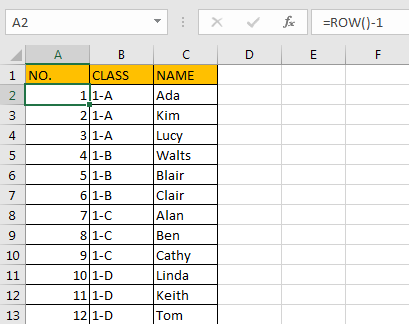Please notice that if there is no header in table and sequence number is started in A1, you can just enter =ROW() here.

Step 2: Create table for this table. Select this table, click Insert tab in ribbon, click Table.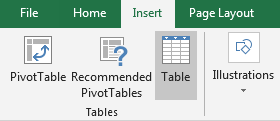Verify that Create Table dialog pops up. As table range is already selected, so \$A\$1:\$C\$13 is auto selected, and option ‘My table has headers’ is checked by default. Click OK.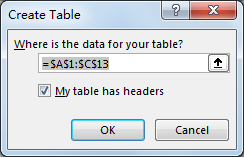Verify that table is created and table style is updated. Now, after creating table, sequence number will be automatically updated.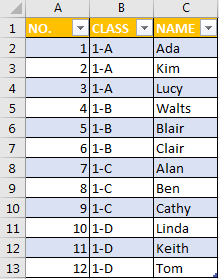Step 3: Try to insert a new row between number 6 and 7.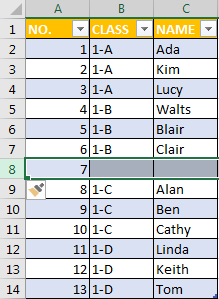Verify that sequence number is updated automatically.

Step 4: Try to delete an existing record.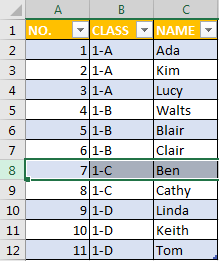Verify that sequence number is updated automatically.

## Method 2: Update Sequence Number for Rows Automatically by VBA Code

Notice: If you want to only delete row from table and then automatically update sequence number, you can also edit VBA code to implement this.

Step 1: On current visible worksheet, right click on sheet name tab to load Sheet management menu. Select View Code, Microsoft Visual Basic for Applications window pops up.

Or you can enter Microsoft Visual Basic for Applications window via Developer->Visual Basic. You can also press Alt + F11 keys simultaneously to open it.

Step 2: In Microsoft Visual Basic for Applications window, enter below code:

```Private Sub Worksheet_Change(ByVal Target As Range)

Dim i As Integer

i = 2

Application.EnableEvents = False

For i = 2 To 13

Range("A" & i).Value = i - 1

Next

Range("A13").Value = ""

Application.EnableEvents = True

End Sub```